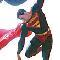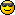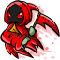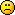# Math Is Fun Forum

Discussion about math, puzzles, games and fun.   Useful symbols: ÷ × ½ √ ∞ ≠ ≤ ≥ ≈ ⇒ ± ∈ Δ θ ∴ ∑ ∫  π  -¹ ² ³ °

You are not logged in.

## #1 2005-03-26 01:11:16

Member
Registered: 2005-03-26
Posts: 2

### On sets and power sets

On P(Z*) > Z*

General:

We say that B > A if A not= B but there is an injection between A and subset of B .

Theorem: |P(Z*)| > |Z*|

Proof:

Step 1: (injection between set A and subset of set B ):

Let us define injection between each member of Z* to each member of P(Z*), which includes a singleton as its content.

We define f(z) where f(z)={z}, for example: 0 <--> {0} , 1 <--> {1} , 2 <--> {2}, and so on.

At this stage we can say that |P(Z*)| >= |Z*| .

Step 2:

Suppose there exists a bijection between P(Z*) and Z* .

There exists member S in P(Z*) which is consists of ALL members of Z* that are not themselves included in the P(Z*) members which they are mapped to, for example:

0 <--> {0,1} , 1 <--> {10,11,12} , 2 <-->{5,6} , 3 <--> {3,4,5} , 4 <--> {8,9},

In this example S ={1,2,4,}.

|Z*|=aleph0   ,    |P(Z*)|=2^aleph0

Bucause {} does not exist in Z*, but {} exists in P(Z*), then S has at least one member of Z*, for example:

z <--> {}

In this example S ={z}.

Now, let us say that there exist some member in Z* (let us call it t ) which is mapped with S (t <--> S ).

In this case we can ask: is t in S or t not in S ?

Options:

1) t in S , but by S definition t cannot be in S .

2) t not in S , in this case by S definition, t must be in S , but by (1) t cant be in S .

And so on, and so on.

Therefore, there cannot be a bijection between P(Z*) and Z* and we can conclude that |P(Z*)| > |Z*| .

Q.E.D

A new point of view of this proof:

By using the empty set (with the Von Neumann Hierarchy), we can construct the set of Z* numbers {0,1,2,3,...}:

0 = |{ }| (notation = {})

1 = |{{ }}| (notation = {0})

2 = |{{ },{{ }}}| (notation = {0,1})

3 = |{{ },{{ }},{{ },{{ }}}}| (notation = {0,1,2})

...

So, through this point of view the atom is {} and the Z* numbers are structural|quantitative combinations of {}.

We have here an Hierarchy of infinitely many complexity levels, starting from {}.

If {} does not exist then we have no system to research, therefore we can learn
that in the base of this number system, there is the idea of dependency, which can be translated to the idea of the power of existence.

Let us look at Cantor's proof from this point of view.

As we all know, any set includes only unique members or no members at all.

Now, let us examine options 1 and 2 again.

Option 1:

All members which included in S , are different from each other.

Any member of Z* can be mapped with some member of P(Z*), once and only once.

Therefore t is different from each member in S, therefore t  MUST BE INCLUDED in S.

(S exists iff t is out of the scope of Cantor's definition. it means that the word ALL is omitted from S definition).

Therefore S cannot be defined, and we cannot check our assumption in step 2 of Cantor's proof.

Option 2:

If we want to keep S as an existing member, we MUST NOT INCLUDE  t in S .

(S exists iff t is out of the scope of Cantor's definition. it means that the word ALL is omitted from S definition).

In this case we also see that S cannot be defined, and again we cannot check our assumption in step 2 of Cantor's proof.

Therefore we cannot ask nor answer to anything that is connected to member S, because S  definition cannot exist, without any connection to the map result between  Z*  and  P(Z*).

The general idea behind this point of view is the power of existence of member t and member S .

More you simple less you depended, therefore more exist.

I'll write S definition again, in an informal way:

There exists member S in P(Z*) which is consists of ALL members of Z* that are not themselves included in the P(Z*) members which they are mapped to, for example:

0 <--> {0,1} , 1 <--> {10,11,12} , 2 <-->{5,6} , 3 <--> {3,4,5} , 4 <--> {8,9},

In this example S ={1,2,4,}.

Now, the power of existence of Z* members is bigger than the power of existence of P(Z*) members.

Member t, which is some arbitrary member of Z* (and mapped with S), is simpler than S, which is a member of P(Z*) .

Member S existence depends on objects like t , therefore we have to check S by t as we did here, and not t by S as Cantor did.

Conclusion: Cantor did not prove that |P(Z*)| > |Z*| because the definition  S={z in Z* such that z is not in f(z)} cannot exist because  P(Z*) cannot exist without Z*.

--------------------------------------------------------------------------------

{{}} can exist only if {} is nested in another {}.

In other words, there is a hierarchy of dependency of composed sets in non-composed sets, and this is exactly the deep meaning of the word hierarchy in Von Neumann Hierarchy, which its unique nested structure is based on 3 ZF axioms:

ZF pair axiom, ZF sum axiom and ZF infinity axiom.

Pair and sum axioms are its recursive side and infinity axiom is its inductive side.

In other words, there is no deep thing here but some arbitrary way to construct nested sets, by using the empty set as their building block, for example:

If → means implies then {} → {{}} → {{},{{}}} → {{},{{}},{{},{{}}}} etc. where the existence of the more composed element depends on the existence of the less composed element.

Also a non-nested collection is simpler than a nested collection, even if they have the same cardinal, for example:

|{{}}| is the same as |{{}}|
|{{},{}}| is simpler than |{{},{{}}}|
|{{},{},{}}| is simpler than |{{},{{}},{{},{{}}}}|
|{{},{},{},{}}| is simpler than |{{},{{}},{{},{{}}},{{},{{}},{{},{{}}}}}|

etc.

Simplicity is one of the most powerful attitudes that can be used in order to understand fine concepts, and I used simplicity in order to research non-empty finite and non-finite collections.

The simplest way to define the unique cardinal of each n in N, is based on the quantity of the empty sets that can be found within each n.

For example:If we examine the 1-1 mapping between each N member and each member of a proper subset of N (notated as S) of even members, we realize that set S is nothing but a particular way to pack the empty sets, which is based on a successor of exactly two empty sets.

Let a successor be the result of card(nth n)-card(nth-1 n) where (nth-1 n)<(nth n).

If nth-1=0 then n has no successor.

Actually we can define ordered non-finite sets of N members, which are different from each other by the sequences of their successors.

But we have to understand that no matter what combination we use, it does not change the 1-1 mapping between a collection of infinitely many empty sets, into itself, for example:If we take the above example of vertical collections of N members, which are constructed by empty sets, and order them in a  horizontal way, we clearly realize that Peano or ZF axioms ignore the empty sets themselves and use a 1-1 mapping between N members, which are actually constructed by packs of empty sets.

The Cantorean 1-1 mapping ignores the level of empty sets, for example:By ignoring the most fundamental level of the empty sets, we can get the illusion that {2,4,6,8,...} is a proper subset of {1,2,3,4,...}.

When we ignore the packed level of both {2,4,6,8,...} and {1,2,3,4,...} and define 1-1 mapping between the empty sets that are included within each natural number, we realize that we actually deal with the same non-finite collection of empty sets.

Furthermore, from this basic level, we also realize that a non-finite collection has no identity map because its 1-1 mapping cannot be satisfied.

In order to understand why, please look at:

http://www.geocities.com/complementaryt … cessor.pdf

Offline

## #2 2005-03-26 09:15:24

MathsIsFunRegistered: 2005-01-21
Posts: 7,711

### Re: On sets and power sets

Thank you, DoronShadmi.  Can you tell me the background of this?  And more about yourself?

"The physicists defer only to mathematicians, and the mathematicians defer only to God ..."  - Leon M. Lederman

Offline

## #3 2005-05-30 23:24:14

Mr T
MemberRegistered: 2005-03-30
Posts: 1,012

### Re: On sets and power sets

i think not

I come back stronger than a powered-up Pac-ManI bought a large popcorn @ the cinema the other day, it was pretty big...some might even say it was "largeFatboy Slim is a LegendOffline

## #4 2005-06-01 10:49:46

munt
Member
Registered: 2005-06-01
Posts: 7

### Re: On sets and power sets

MAtshsS is thse r00xx0rzzfOffline

## #5 2005-06-01 10:59:17

Zach
MemberRegistered: 2005-03-23
Posts: 2,075

### Re: On sets and power sets

That's a pretty funny picture.

Boy let me tell you what:
I bet you didn't know it, but I'm a fiddle player too.
And if you'd care to take a dare, I'll make a bet with you.

Offline

## #6 2005-07-08 06:05:56

Roraborealis
MemberRegistered: 2005-03-17
Posts: 1,594

### Re: On sets and power sets

That's crueltySchool is practice for the future. Practice makes perfect. But - nobody's perfect, so why practice?

Offline